Next: Zero Energy n-p scattering Up: PHYS 560: Lectures During Previous: Scattering in a Central

# Low-Energy S-Wave Scattering

Let us familiarize ourselves with these scattering amplitudes by examining low energy scattering in the S-wave. In this scenario we have only one partial wave contributing (obviously) with a phase shift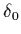. The cross section for S-wave scattering is then given in terms of this one phase shift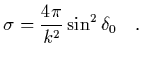(21)

It is clear that for a finite range potential, such as the strong interaction potential between nucleons, the cross section is finite for all energies, and hence the phase shiftmust vanish at least as fast as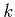in order, so that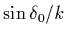is finite. Hence for smallthe phase shift will behave as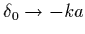, where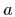is some constant with dimensions of length (to compensate the dimensions of mass of k). In this limit one can also replace the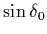with, to yield a cross section in terms of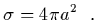(22)

The constantis called the scattering length.

In the limit of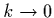as we have been considering above, the solution to the schrodinger equation in the region where the potential vanishes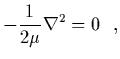(23)

is a straight line. We already know this as the solution for anyis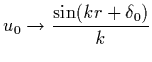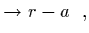(24)

in the low momentum regime. We see that the scattering lengthis the radius at which the asymptotic (straight line) solution vanishes. This is, of course, a limit of the incident oscillatory waves.

One of the things we should learn from this is that all we see in the low energy (compared to the inverse radius of the potential) is the asymptotic behaviour of the wavefunction characterized by one constant, the scattering length. This is a nontrivial construction from the potential itself and the wavefunction of the state. We see that for momenta much less than the inverse radius of the potential the scattering length is sufficient to describe all of the interactions. This goes back to our original ideas about short distance physics being encoded in the constants of the low energy theory. It is clear that by measuring the scattering length of a system alone we cannot reconstruct the potential uniquely. There are infinitely many different shapes, depths and ranges of potentials that will reproduce a single scattering length.

Let us consider the system I have shown in the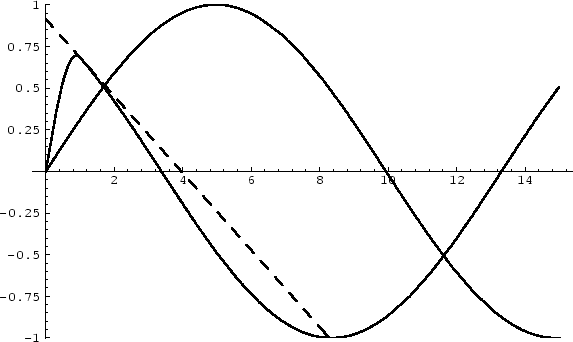which corresponds to a finite square well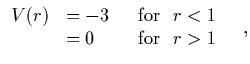(25)

in which a system of reduced mass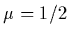moves. The oscillatory curves correspond to the wavefunctions for particles incident upon the potential of energy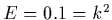for. One is for the system with the potential given above while the other is that for a vanishing potential. I have also shown the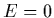wavefunction for the potential. We see that the oscillatory wavefunction intercept with the x-axis is tending to the scattering length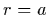defined by theintercept, shown by the straight line for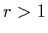. The phase shift (divided by) corresponds to the difference between the intercepts with the x-axis, i.e. the difference between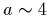and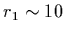. This would say that the scattering amplitude is given by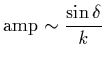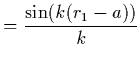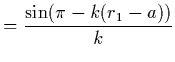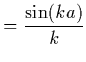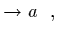(26)

where we used the fact that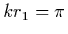by construction.

It is important to notice that for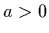the wavefunction inside this potential must have just turned over to have a negative derivative at the boundary. This would indicate that a bound state exists for this potential. In order for us to arrive at this conclusion we see that the existence of a bound state requires that the wavefunction at the boundary have a negative derivative so that we can match onto an exponentially falling wavefunction outside the potential. Such a fall off is characteristic of a state with negative energy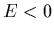, and is localized about the centre of the potential. We will return to this later on. Also, we note that if the scattering length were negative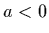, then the wavefunction would have a positive derivative at the boundary of the potential. This indicates that there is not a loosely bound (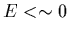) state for this system.

So far we have considered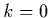scattering and found that a single parameter can be identified, the scattering length, and we have discussed what this means in terms of wavefunctions and cross sections. However, when we move away fromscattering and ask about the cross section for non-zero momenta but still much smaller than the inverse radius, then we expect to be able to perform a taylor expansion on the scattering amplitude, or some quantity related to the scattering amplitude. We have observed in our previous discussions that we can "encode" (in some complicated way) the potential and wavefunction in this region by the phase shift of the eigenstates of the potential. We expect that the dependence on small momenta (compared with the potential range) can also be encoded by this phase shift. We therefore wish to form a derivative expansion of the phase shift, or some function of the phase shift, if it is more appropriate. Let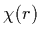be the asymptotic form ofas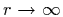, where the radial wavefunction is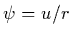. We have already seen that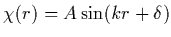and we can form the quantity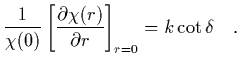(27)

We choose to perform the taylor expansion of this object in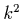. It is quadratic inbecause we are perturbing the solution to the schrodinger equation about, andappears quadratically. We define the effective range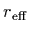by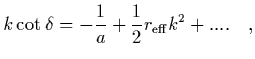(28)

where thelimit coincides with our previous definition of the scattering length.

Before we proceed, lets look at this object a slightly different way. Starting with the scattering amplitude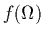for an S-wave interaction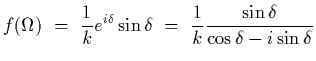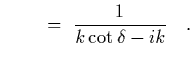(29)

Therefore, if we understand the object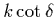, then we understand the scattering amplitude, and hence the cross section. Many years ago, Schwinger and others examined the properties of. For a finite range potential, range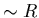, the quantityhas a power series expansion about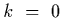that converges for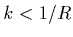. We write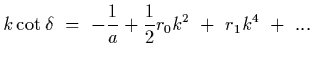(30)

It is easy to see that this correctly reproduces the smallbehaviour of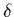. In fact one can show, as may already be obvious from the figure that the scatterinbg length is not bounded by the range of the potential, but the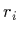are set by the range of the potential, i.e.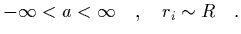(31)

We will spend some time discussing where the following numbers come from, but it is found that from neutron proton scattering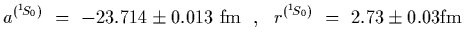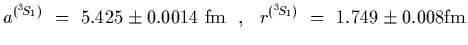(32)

An interesting feature of, is that when the entire denominator ofvanishes, the scattering amplitude becomes infinite, corresponding to the existance of a bound state at that location. Therefore, solving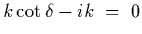determines the location inspace of bound states in either channel. In the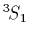channel, this corresponds to the deuteron bound state with a binding energy of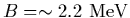. In the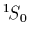channel the pole has positive energy corresponding to a resonance in the scattering amplitude. In fact, the location of the deuteron pole is one of the inputs into the precise determination of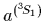and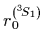.Next: Zero Energy n-p scattering Up: PHYS 560: Lectures During Previous: Scattering in a Central
Martin Savage
1999-10-05